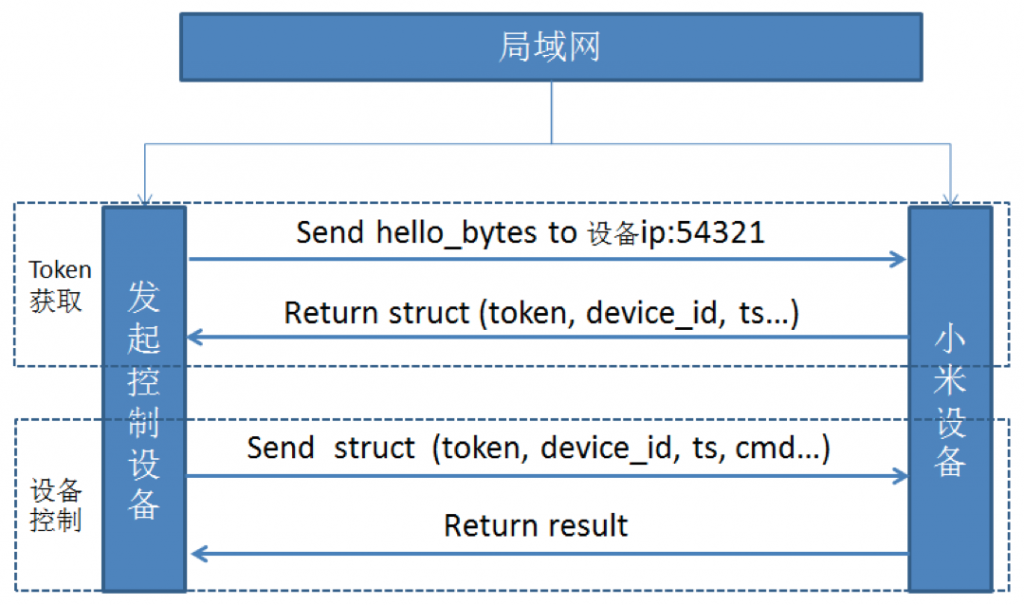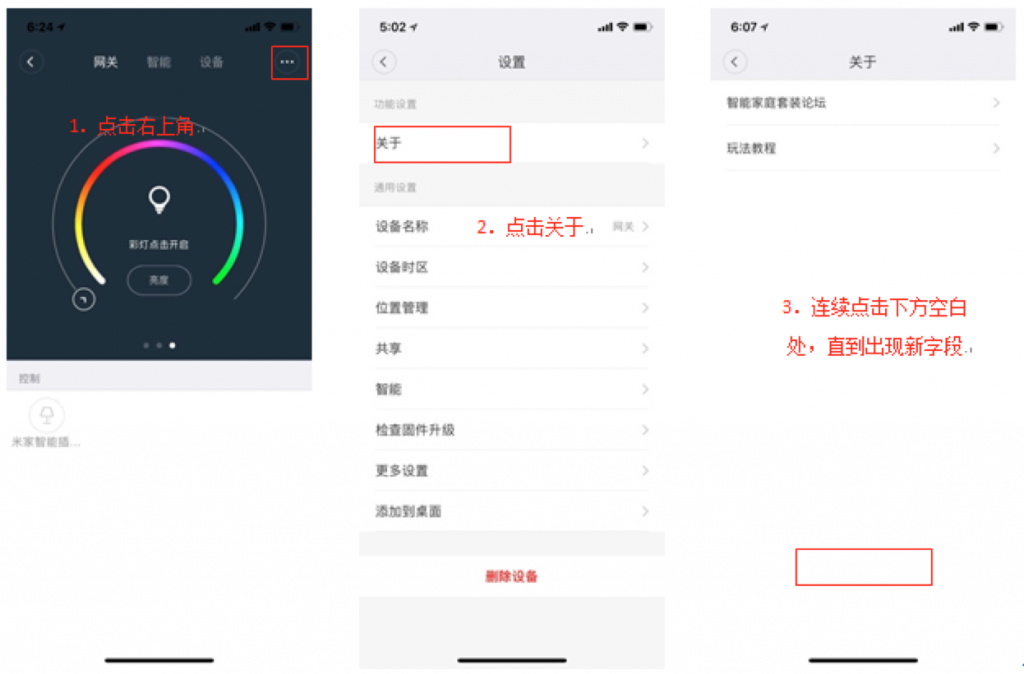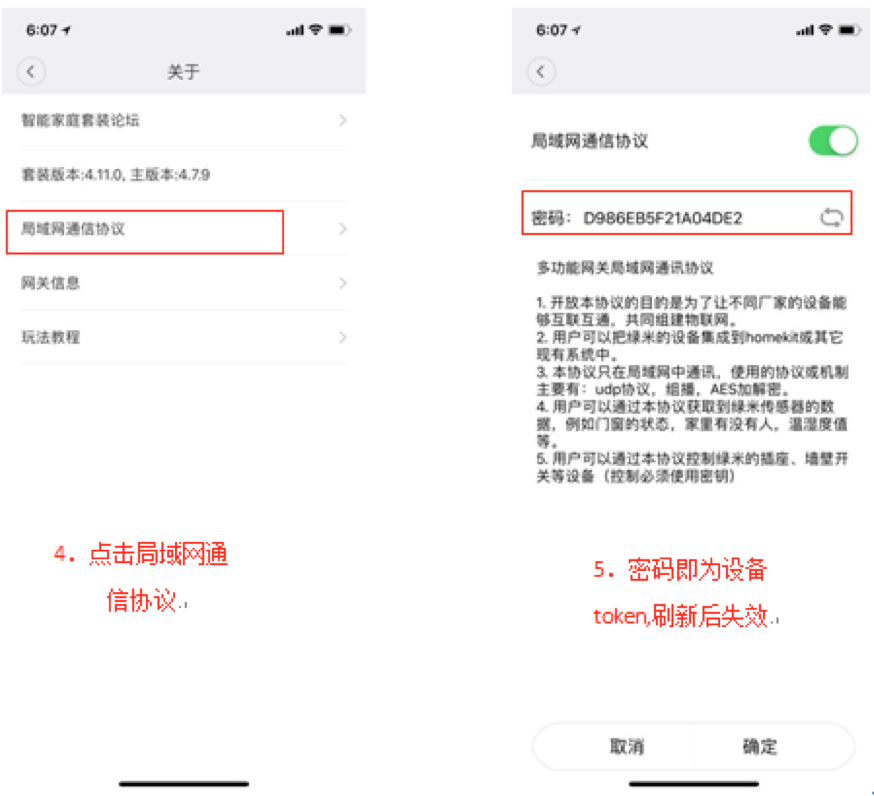## 一、总体流程介绍## 二、小米设备token获取

### 2.1 miio获取token

miio有基于Python实现的库，其Github项目地址为：https://github.com/rytilahti/python-miio。该项目支持所有兼容miio协议的设备，并将设备发现、识别和控制的方法进行了分类。

#### 2.1.1 环境安装

python-miio需要Python3.5以上版本上才能运行，所以首先搭建Python环境。下面，我们在操作系统为Ubuntu的电脑或者树莓派中安装Python3.5：

• 安装5依赖（本机存在的会忽略）
`sudoapt-getinstallbuild-essentiallibsqlite3-devsqlite3bzip2libbz2-devlibssl-devopenssllibgdbm-devliblzma-devlibreadline-devlibncursesw5-dev`
• 编译安装5
```wgethttps://www.python.org/ftp/python/3.5.2/Python-3.5.2.tgz

tarzxvfPython-3.5.2.tgz

cd./Python-3.5.2

./configure--prefix=/usr/bin/python3.5

sudomake

sudomakeinstall
```
• 编译后运行一下5，结果如下证明安装成功
```sean@ubuntu:~/Desktop/week/ProcessAndDeadline\$ python3.5

Python3.5.2(default,Nov232017,16:37:01)

[GCC5.4.020160609]onlinux

Type"help","copyright","credits" or"license" formoreinformation.

>>>```
• 安装miio库，下载库代码到本地并安装
```gitclonehttps://github.com/rytilahti/python-miio

cd python-miio/

python3.5 setup.py install```

#### 2.1.2 通过脚本获取token

• 脚本编写

```#-*-coding:utf8-*-

importcodecs

importsocket

fromprotocolimportMessage

helobytes=bytes.fromhex('21310020ffffffffffffffffffffffffffffffffffffffffffffffffffffffff')

s=socket.socket(socket.AF_INET,socket.SOCK_DGRAM)

s.sendto(helobytes,('192.168.42.17',54321))#插座ip，端口54321

data,addr=s.recvfrom(1024)

m=Message.parse(data)

tok=codecs.encode(m.checksum,'hex')

print(m)

print(tok)```
• 运行：5 miio_test.py

```root@raspberrypi:~/python-miio/miio#python3.5miio_test.py

Container:

data=Container:

offset2=32

offset1=32

length=0

value=  (total0)

data=  (total0)

header=Container:

offset2=16

offset1=0

length=16

value=Container:

length=32

unknown=0

device_id=\x03\xa9\x84\xb6(total4)

ts=1970-01-2222:10:05

data=!1\x00\x00\x00\x00\x00\x03\xa9\x84\xb6\x00\x1c\xe7=(total16)

checksum=\x1d\x06\x16\x85\x80b\xb8\xf86\xeb\xca\xcc\x98\xe6-\xd2(total16)

b'1d0616858062b8f836ebcacc98e62dd2'```

### 2.2 从米家app获取token### 2.3 从数据库获取token

• 准备一部获取root权限的安卓手机
• 安装米家app并登录账号
• 进入/data/data/com.xiaomi.smarthome/databases/
• 拷贝db，下载到电脑
• 前往网站（http://miio2.yinhh.com/），上传db，点击提交，即可获得token。

## 三、控制小米WiFi插座

### 3.1 控制脚本编写

```ts=m.header.value.ts+datetime.timedelta(seconds=1)

cmd={'id':1,'method':'set_power','params':['on']}

header={'length':0,'unknown':0x00000000,

'device_id':device_id,'ts':ts}

msg={'data':{'value':cmd},

'header':{'value':header},

'checksum':0}```

token为获取到的设备token；

device_id为获取token返回结构中的device_id字段；

ts是一个时间结构，控制传的ts的需要在获取到ts基础上加1秒；

cmd中的method包括：set_power(控制开关)、get_prop(获取状态)，控制的params是[‘on’]/ [‘off’]，获取状态的params是[‘power’, ‘temperature’]

```m0=Message.build(msg,token=m.checksum)

s=socket.socket(socket.AF_INET,socket.SOCK_DGRAM)

s.sendto(m0,('192.168.42.17',54321))

data,addr=s.recvfrom(1024)

m1=Message.parse(data,token=tok)

print(m1)```

### 3.2 执行控制脚本

```root@raspberrypi:~/python-miio/miio#python3.5miio_test.py

Container:

data=Container:

value={'id':1,'result':['ok']}

data=\x03\x88T\x86\x1a\xbd\xb5\xb24.\xdcm\xdb\xc3\xb4\xdb\x0e7\x80JR\x0e\xda\xa987\x91Q\xd0\xee\x9bV(total32)

offset2=64

offset1=32

length=32

header=Container:

value=Container:

length=64

unknown=0

device_id=\x03\xa9\x84\xb6(total4)

ts=1970-01-2220:40:38

data=!1\x00@\x00\x00\x00\x00\x03\xa9\x84\xb6\x00\x1c\xd2F(total16)

offset2=16

offset1=0

length=16

checksum=\xfc\xe2\r\x8b\xd9\xb6\x1d\xfda\xd5\x11\x04\xe1b\xbe\xfd(total16)```

## 四、总结

Spread the word. Share this post!